#Function Repository Resource:

# SquareZigzagPoints

Lattice points in a square in zigzag order from the bottom-left to the top-right corner

Contributed by: George Beck
 ResourceFunction["SquareZigzagPoints"][n] gets all the points in the square with opposite corners {1,1} to {n,n} in zigzag order.

## Details and Options

The square has side length n-1.
In zigzag order, the points form a path N, SE, E, NW, N, ….

## Examples

### Basic Examples

This shows how the points are ordered in the simplest case:

 In:=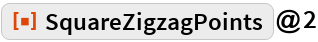Out=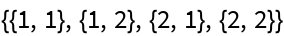In:=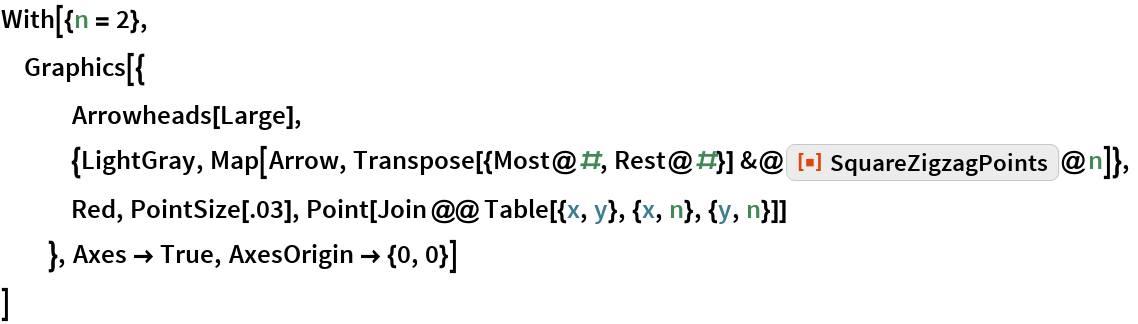Out=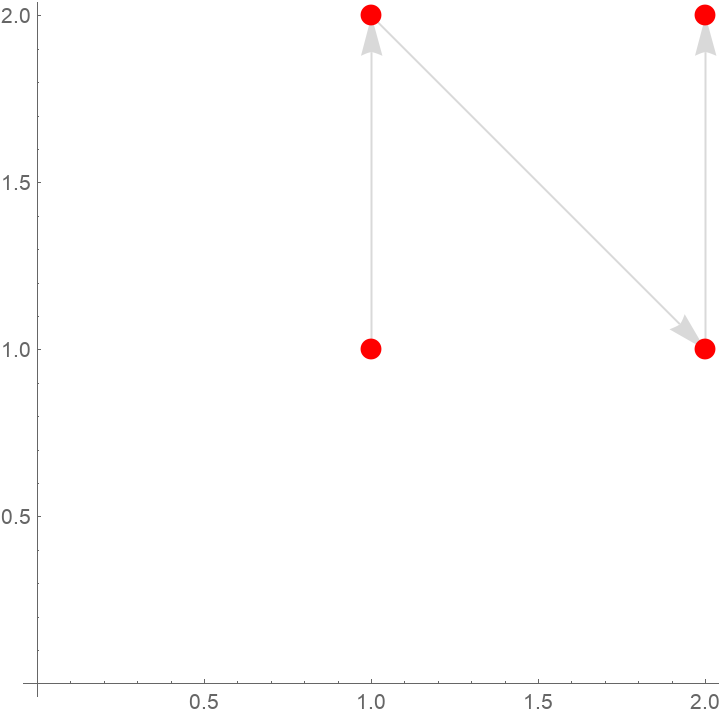A larger example:

 In:=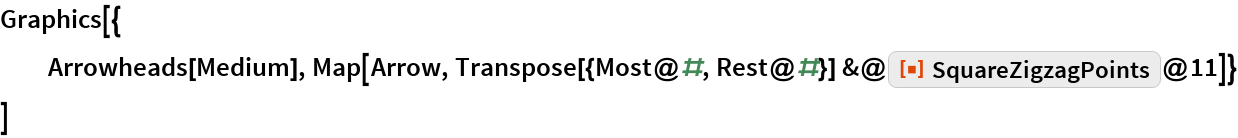Out=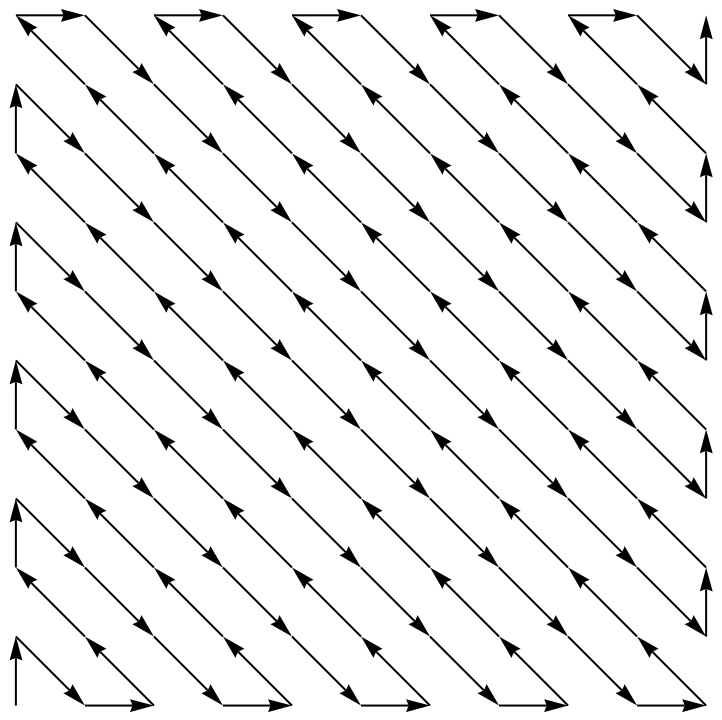### Scope

This starts at the bottom right:

 In:=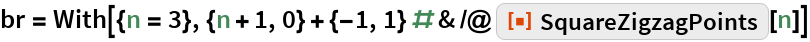Out=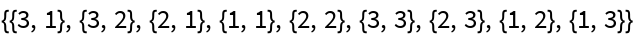This shows the path:

 In:=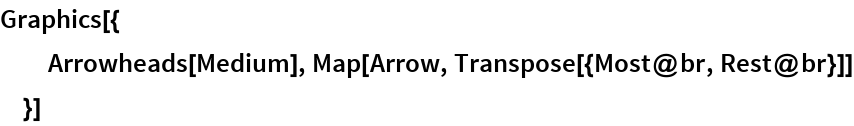Out=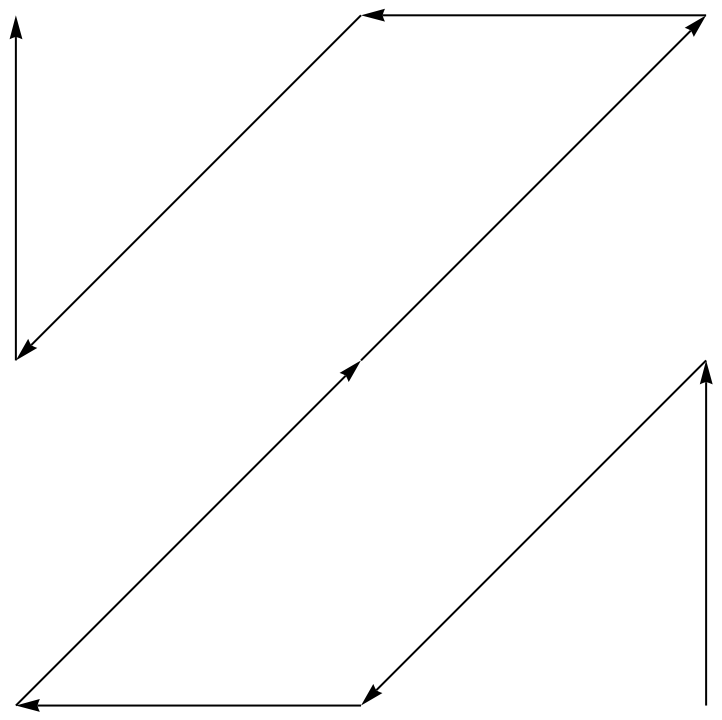This starts horizontally at the bottom right:

 In:=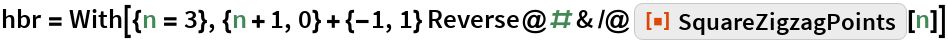Out=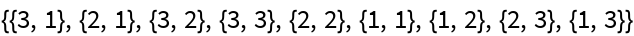This shows the path:

 In:=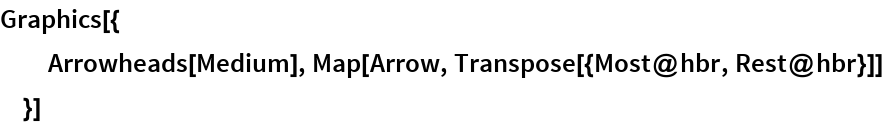Out=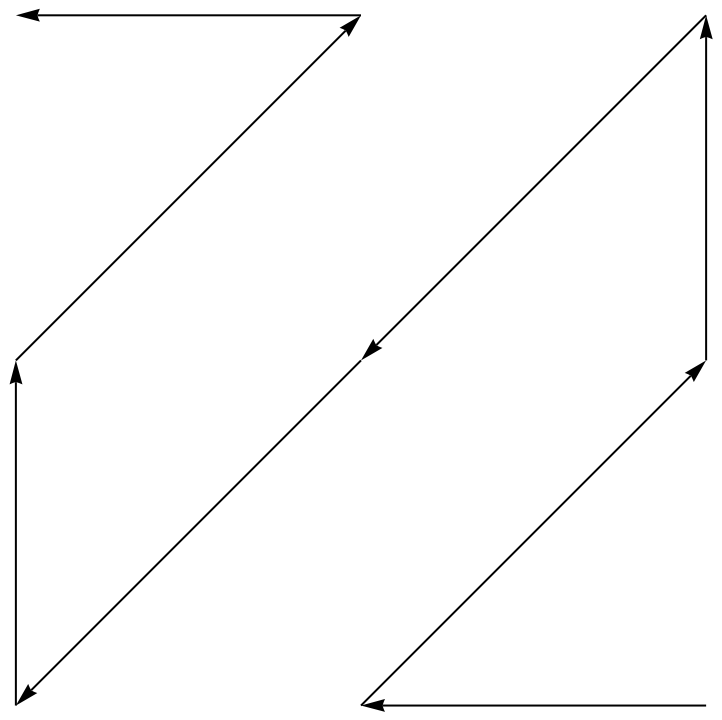## Requirements

Wolfram Language 11.3 (March 2018) or above